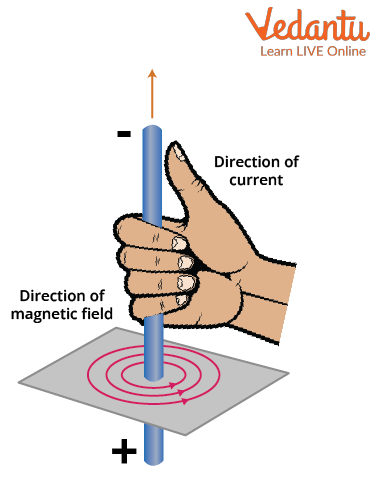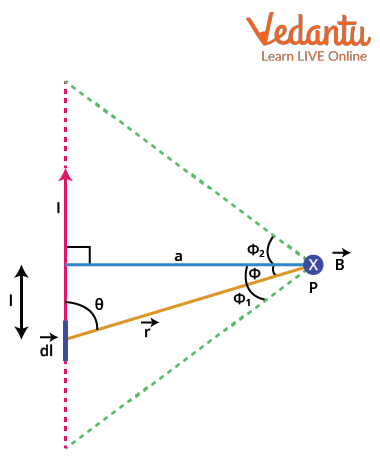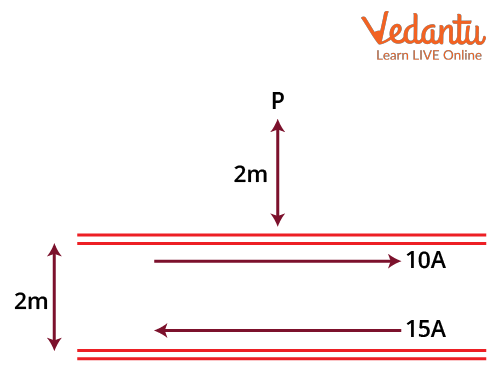Courses
Courses for Kids
Free study material
Free LIVE classes
More

# Magnetic Field Due to Straight Wire and Its Formula for JEELIVE
Join Vedantu’s FREE Mastercalss

## An Introduction to Magnetic Field due to Straight Wire

Magnetic fields are produced when current passes through a wire or a loop. Moreover, a strong current is required to produce a strong magnetic field. A long straight wire carrying current will produce a magnetic field. If we place another current-carrying wire in its vicinity, it experiences a force that is produced by the first current-carrying wire.

We derive this force on the wire using Biot's Savart's Law. Biot Savart’s law states the relationship between a current-carrying wire and a point P placed at a distance r from the wire. The magnitude of this magnetic field is inversely proportional to the square of the distance of wire to the point P kept at distance r.

## Magnetic Field Due to Straight Wire Formula

The magnetic field due to straight wire is produced by moving charges. The direction of this current can be found out from Maxwell's right thumb rule.Right Hand thumb Rule

Right Hand Thumb Rule: The right hand thumb rule states that when we curl our fingers in the direction of the magnetic field, the direction of the thumb gives the direction of the current. Inversely, it can also be stated that if the thumb represents the direction of current, then the fingers denote the direction of the magnetic field.

Assuming a long straight current carrying a wire of magnitude I, the magnetic field (B) created by the conductor is formed around it. The magnetic field also further depends on the distance of the wire. Thus, the magnetic field created by the current-carrying wire is denoted as the ratio of the product of magnetic permeability and current to its distance from the wire. Mathematically, it can be denoted as :

$B=\dfrac{\mu{ }_{o}I}{2 \pi r}$

where

$\mu$ is the permeability of free space or $4\pi\times10^{-7}~\left(\dfrac{T\cdot m}{A}\right)$.

B is the magnetic field measure in tesla.

I is the current intensity flowing in the long wire.

r is the distance of the magnetic field from the wire.

## Magnetic Field Due to Straight Wire Derivation

Suppose we assume a point P at a distance r from a long straight wire carrying current I.Magnetic Field due to a Long Straight Wire

Now, according to Biot Savart’s Law, the magnetic field of a point P placed at a distance r is

$\mathrm{d} B=\dfrac{\mu_{\theta} I d l \sin (\theta)}{4 \pi r^{2}}$

From the figure, it can be seen that

\begin{align} &r=\sqrt{a^{2}+l^{2}} \\ &\sin \theta=\dfrac{r}{\sqrt{a^{2}+l^{2}}} \end{align}

We need to calculate the magnetic field for the entire conductor. We can find this by integrating the equation for half the wire and multiplying it by 2. Then, the limits of the integral will be from 0 to infinity.

The integration gives

$\int d B=2 \times \int_{0}^{\infty} \dfrac{\mu_{0} I}{4 \pi} \dfrac{1}{\left(\sqrt{r^{2}+l^{2}}\right)^{2}} \dfrac{r}{\sqrt{r^{2}+l^{2}}} \mathrm{~d} l$

$B=\dfrac{\mu_{0} I}{2 \pi} \int_{0}^{\infty} \dfrac{r}{\left(r^{2}+l^{2}\right)^{3 / 2}} \mathrm{~d} l$

$B=\dfrac{\mu_{0} I}{2 \pi r}\left[\dfrac{l}{\left(r^{2}+l^{2}\right)^{1 / 2}}\right]_{0}^{\infty}$

\begin{align} &B=\dfrac{\mu_{0} I}{2 \pi r}\left[\lim _{l \rightarrow \infty}\dfrac{l}{l\left(\dfrac{r^{2}}{l^{2}}+1\right)^{\dfrac{1}{ 2}}}-0\right] \\ &B=\dfrac{\mu_{0} I}{2 \pi r}\left[\dfrac{1}{\left(\dfrac{r^{2}}{\infty}+1\right)^{\dfrac{1}{ 2}}}-0\right] \\ &B=\dfrac{\mu_{0} I}{2 \pi r}[1-0] \\ &B=\dfrac{\mu_{o} I}{2 \pi r}\end{align}

Thus, the magnetic field due to a long straight current carrying wire is inversely proportional to the distance of a point P from the wire.

### Electric Field Due to Straight Wire

The electric field for a long straight current-carrying wire is denoted as: $E=\dfrac{\lambda}{2 \pi \in{ }_{o}{r}}$

where r is the distance of the electric field from the wire, $\lambda$ is the linear charge density, and $\in_{o}$ is the permittivity whose value is 8.854 $\times$ 10-12 .

This field can be calculated by considering an infinitely long straight wire and then using a cylindrical gaussian surface. The gauss law can then be used to find the electric field of this straight wire.

## Solved Examples on Magnetic Field Due to Straight Wire

Example 1:  A wire of 60 cm in length carries a current I= 4 A. What is the magnetic field at 50 cm from the wire?

Solution:

As derived from above the formula, magnetic field of a straight line is denoted as:

$B=\dfrac{\mu I}{2 \pi r}=\dfrac{4 \pi \times 10^{-7} .4}{(2 \pi \times 0.6 m)}=13.33 \times 10^{-7}$

Thus, the value of the magnetic field comes out to be 13.33 $\times$ 10-7 tesla.

Example 2: A wire of 60 cm in length carries a current I= 3 A. At which distance is the magnetic field 2 $\times$ 10-3 T?

Solution:

As derived from above the formula, magnetic field of a straight line is denoted as:

\begin{align}&r=\dfrac{\mu I}{2 \pi B}=\dfrac{4 \pi \times 10^{-7} \times 3}{2 \pi \times 2 \times 10^{-3}} \\ &r=0.6 \times 10^{-4} \mathrm{~m}=0.06 \mathrm{~mm} \end{align}

Thus, the distance at which the magnetic field is 2 $\times$ 10-3 T is 0.06 mm.

Example 3: Find the electric field at 6m from an infinitely long wire with a linear charge density of 8 x 10-3 $\dfrac{C}{m}$.

Solution:

Given r = 5m and $\lambda$=   8 $\times$ 10-3 cm. Substituting these in the above formula, we get,

\begin{align}E=\dfrac{\lambda}{2 \pi \in{ }_{o}^{r}} \\E=\dfrac{8 \times 10^{-3}}{2 \pi \times 6 \times 8.854 \times 10^{-12}}\\ E=0.047 \times 10^{9}\,N/C \\ \end{align}

Hence, the value of electric field is $0.047 \times 10^{9}\,N/C$.

Example 4:  A straight current-carrying conductor is carrying a current of  10A and another conductor parallel to it carries a current of 15A on the opposite side as shown in the figure below. Find the magnitude of the magnetic field produced by the system at a distance of 2 m.Diagram for the problem

Solution:  The problem has two wires. Now we can find the magnetic field by individual wires and then add them to get the total magnetic field. Let the magnetic field by wire carrying 10A current be B1. We can write B1 as

\begin{align} &B_{1}=\dfrac{\mu_{o}{I}_1}{2 \pi r_{1}} \\ &B_{1}=\dfrac{2 \mu_{o} \times 10}{4 \pi \times 2} \\ &B_{1}=10^{-7} \times 10 \\ &B_{1}=10^{-6} \mathrm{~T} \end{align}

Similarly, the magnetic force B2 by second wire carrying current 15 A and at distance 4m from P can be given as

\begin{align} &B_{2}=\dfrac{\mu_{0} I_{2}}{2 \pi r_{2}} \\ &B_{2}=\dfrac{2 \mu_{0} \times 15}{4 \pi \times 4} \\ &B_{2}=10^{-7} \times \dfrac{15}{2} \\ &B_{2}=0.75 \times 10^{-6} \mathrm{~T} \end{align}

Now, both the wires have opposite directions, so the total magnetic field will be given by the difference of their individual magnetic fields. Thus, the total  magnetic field B at point P can be written as

\begin{align} &B=B_{1}-B_{2} \\ &B=10^{-6}-0.75 \times 10^{-6} \\ &B=0.25 \times 10^{-6} \mathrm{~T} \end{align}

So, the magnetic field by both wires at point P is 0.25$\times$ 10-6 T.

## Conclusion

The article presents a thorough walkthrough of the magnetic field for a long infinite straight current carrying wire. The magnetic field is created due to current and its strength depends on the magnitude of the current. The magnetic field for a long straight infinite current carrying wire is inversely proportional to its distance from the wire. Electric field on the other hand  is denoted as $E=\dfrac{\lambda}{2 \pi \in{ }_{o}{r}}$, which is again inversely proportional to the distance of the point from the wire.

Last updated date: 20th Sep 2023
Total views: 132.3k
Views today: 1.32k

## FAQs on Magnetic Field Due to Straight Wire and Its Formula for JEE

1. What is the direction of force created by a conductor carrying current?

The direction of the force produced by a long wire is given by the right hand  thumb rule. Stretch the thumb, middle, and index finger of your right hand perpendicular to one another. The index finger here will denote the direction of the velocity of the conductor, the magnetic field denotes the direction of the magnetic field, and the thumb depicts the force acting on the conductor. It must be noted that this rule is applicable for a conductor moving in a magnetic field to find the direction of force acting on it.

2. What is the weightage of the magnetic field due to a straight wire in JEE?

Magnetic field due to straight wire comes under the chapter magnetic effects of current and magnetism. It is an extremely important topic and has a high chance of being asked in the exam. A variety of questions can be framed from magnetic fields due to straight wire and so you need to practise as many problems as possible. The chapter on magnetic effects of current and magnetism has a weightage of about 6.6%  in and at least 2 questions are asked every year from this topic.